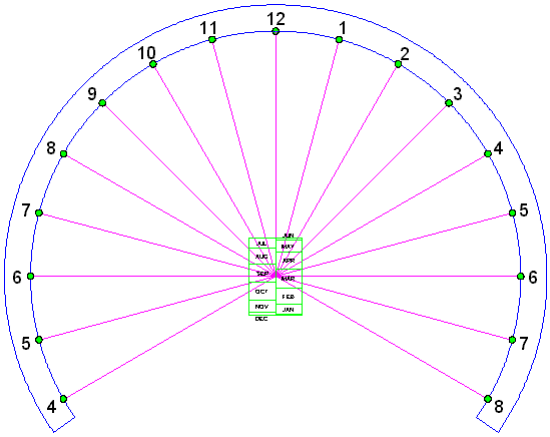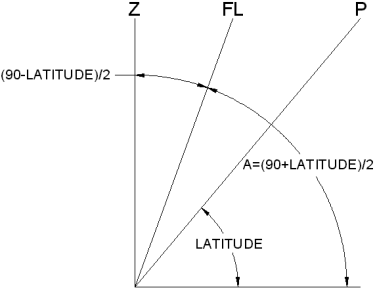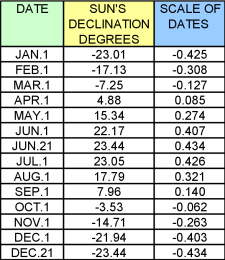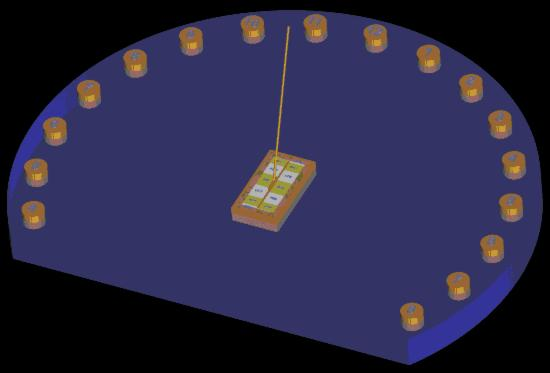The Sundial Primer created by Carl SabanskiThe Sundial Primer Index
 Foster-Lambert Sundial Foster-Lambert Sundial: a form of equatorial projection dial, with the projection arranged to produce a circular ring of equiangular hour points. Analemmatic Sundial: sundials consisting of hour points (rather than hour lines) laid round an ellipse, and a movable gnomon perpendicular to the dial plane. It may be on any plane, but the most usual form is horizontal. In the horizontal version of the dial, gnomon position lies on the straight North-South minor axis, at a point determined by the sun's declination (i.e. the date). The analemmatic dial may be regarded as a projection of the universal equatorial ring dial. Azimuthal or Azimuth Sundial: any dial which uses the sun's azimuth for indicating the time. It usually needs to be aligned N-S, and has a vertical style (if it has no dependence on altitude).Declination (of the Sun): the angular distance of the Sun above or below the celestial equator. Its value follows an annual sine wave like curve, varying between 0° at the equinoxes and ±23.4° (approx.) at the solstices. It has positive values when the Sun is above the celestial equator (summer in the Northern hemisphere) and negative when below. Hour Point: a point on a dial plane indicating the crossing of the gnomon's shadow at a particular time. Hour points replace hour lines on dials where the shadow edge does not pass through the dial centre. The Foster-Lambert sundial is a form of the analemmatic sundial. The sundial presented here is a horizontal azimuth sundial with a moveable gnomon. There are no hour lines but hour points. The hour points are located on the circumference of a circle and are equiangular. For this to occur the gnomon is set at an angle. To indicate the time the gnomon is placed on a scale of dates at a specific location for the current date. The determination of the hour points on the circumference of the circle is simply a matter of spacing them 15º for every hour, 7.5º for 30 minutes, 3.75º for 15 minutes, etc. To calculate the layout of the scale of dates you will need to know the sun's declination on particular dates for each month. To determine the angle of the gnomon requires that you know the latitude (ø) where the sundial is to be located. Figure 1 illustrates a Foster-Lambert sundial with its scale of dates.Figure 1: Foster-Lambert Sundial (CAD) The gnomon of a Foster-Lambert sundial is not perpendicular to the dial plate but is slanted. As illustrated in Figure 2 the gnomon lies half way between a polar pointing gnomon "P" and a vertical gnomon "Z". Its position can be calculated in two ways but the following calculation of the angle "A" relative to the horizontal dial plate is used in the equations given later.A = (90 + ø) / 2 where ø is the latitude. The span of the gnomon's angle "A" is from 45º at a latitude of 0º to 90º at a latitude of 90º. The gnomon lies on the meridian line.Figure 2: Foster-Lambert Sundial Gnomon (CAD) Figure 3 illustrates the design layout for the sundial. The first step is to select a suitable radius "R" for the circle on which the hour points will be positioned. Place the desired number of hour points around the circumference of the circle based on the fact they are equiangular and every full hour is separated by 15º. The meridian line is a vertical line passing through the centre "C" of the sundial and the local apparent noon hour point. If a line is drawn relative to the meridian line at an angle equal to "90-A" starting from the noon hour point and terminating at the horizontal line, it will make the "A" relative to the horizontal line. It will also establish the position of "E". The position of "E" from the centre "C" is calculated as follows:E = R / tan A = R * tan (90 - A) The position of "E" will range from "R" at a latitude of 0º to 0 at a latitude of 90º.Figure 3: Foster-Lambert Sundial Layout (CAD) The next step is to establish the scale of dates. This is the scale used to determine the position of the gnomon on a given day of the year. The scale points are determined for the first day of each month and for the two extremes at the time of the solstices. To do this, the sun's declination is required for each of these dates. The values used were obtained from "The Dialist's Companion". The point "E" is necessary to establish the scale of dates as each scale point is determined by finding the intersection of a line from "E" to the meridian line drawn at an angle equal to the sun's declination on the desired date. The distance from the sundial's centre "C" to the intersection point "Y" on the meridian line for any desired date is calculated as follows:Y = E * tan (dec)where "dec" is the sun's declination.Figure 3 illustrates how the scale points are found for the two solstices. In Table 1 the calculation is performed for a sundial with "E" set to "1". For any value of "E" multiply by the factors in Table 1 to obtain the scale points.Table 1: Foster-Lambert Sundial Scale of DatesThe longest length for scale of dates will be "0.434*R" at a latitude of 0º  and will decrease to 0 at a latitude of 90º.The layout of the scale of dates begins at the dial centre "C". Positive numbers are measured up the meridian line and negative numbers down the meridian line. Figure 4 illustrates a layout for the scale of dates.Figure 4: Scale of Dates (CAD)Because the hour points are equiangular it is quite simple to correct for the Equation of Time, longitude and daylight saving time. The dial needs to be designed so the hour points can rotate about the centre "C" independently of the remainder of the dial plate. The hour points are then rotated to the required correction.The Foster-Lambert sundial can be designed to be an interactive sundial but it would be difficult to use a person as the gnomon. A moveable gnomon at the correct angle would need to be designed. The following images look a bit different because the dial plate is made from glass and you can see through it.For an image complete with shadow click here.